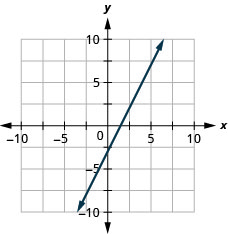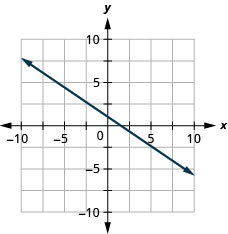# 4.5E: Exercises

•• OpenStax
• OpenStax
$$\newcommand{\vecs}{\overset { \rightharpoonup} {\mathbf{#1}} }$$ $$\newcommand{\vecd}{\overset{-\!-\!\rightharpoonup}{\vphantom{a}\smash {#1}}}$$$$\newcommand{\id}{\mathrm{id}}$$ $$\newcommand{\Span}{\mathrm{span}}$$ $$\newcommand{\kernel}{\mathrm{null}\,}$$ $$\newcommand{\range}{\mathrm{range}\,}$$ $$\newcommand{\RealPart}{\mathrm{Re}}$$ $$\newcommand{\ImaginaryPart}{\mathrm{Im}}$$ $$\newcommand{\Argument}{\mathrm{Arg}}$$ $$\newcommand{\norm}{\| #1 \|}$$ $$\newcommand{\inner}{\langle #1, #2 \rangle}$$ $$\newcommand{\Span}{\mathrm{span}}$$ $$\newcommand{\id}{\mathrm{id}}$$ $$\newcommand{\Span}{\mathrm{span}}$$ $$\newcommand{\kernel}{\mathrm{null}\,}$$ $$\newcommand{\range}{\mathrm{range}\,}$$ $$\newcommand{\RealPart}{\mathrm{Re}}$$ $$\newcommand{\ImaginaryPart}{\mathrm{Im}}$$ $$\newcommand{\Argument}{\mathrm{Arg}}$$ $$\newcommand{\norm}{\| #1 \|}$$ $$\newcommand{\inner}{\langle #1, #2 \rangle}$$ $$\newcommand{\Span}{\mathrm{span}}$$$$\newcommand{\AA}{\unicode[.8,0]{x212B}}$$

## Practice Makes Perfect

Recognize the Relation Between the Graph and the Slope–Intercept Form of an Equation of a Line

In the following exercises, use the graph to find the slope and $$y$$-intercept of each line. Compare the values to the equation $$y=mx+b$$.

##### Exercise $$\PageIndex{1}$$$$y=3x−5$$

##### Exercise $$\PageIndex{2}$$$$y=4x−2$$

slope $$m=4$$ and $$y$$-intercept $$(0,−2)$$

##### Exercise $$\PageIndex{3}$$$$y=−x+4$$

##### Exercise $$\PageIndex{4}$$$$y=−3x+1$$

slope $$m=−3$$ and $$y$$-intercept $$(0,1)$$

##### Exercise $$\PageIndex{5}$$$$y=-\frac{4}{3} x+1$$

##### Exercise $$\PageIndex{6}$$$$y=-\frac{2}{5} x+3$$

slope $$m=-\frac{2}{5}$$ and $$y$$ -intercept $$(0,3)$$

Identify the Slope and $$y$$-Intercept From an Equation of a Line

In the following exercises, identify the slope and $$y$$-intercept of each line.

##### Exercise $$\PageIndex{7}$$

$$y=−7x+3$$

##### Exercise $$\PageIndex{8}$$

$$y=−9x+7$$

$$m = −9$$; $$y$$-intercept: $$(0,7)$$

##### Exercise $$\PageIndex{9}$$

$$y=6x−8$$

##### Exercise $$\PageIndex{10}$$

$$y=4x−10$$

$$m = 4$$; $$y$$-intercept: $$(0,−10)$$

##### Exercise $$\PageIndex{11}$$

$$3x+y=5$$

##### Exercise $$\PageIndex{12}$$

$$4x+y=8$$

$$m = −4\0; \(y$$-intercept: $$(0,8)$$

##### Exercise $$\PageIndex{13}$$

$$6x+4y=12$$

##### Exercise $$\PageIndex{14}$$

$$8x+3y=12$$

$$m = -\frac{8}{3}$$; $$y$$-intercept: $$(0,4)$$

##### Exercise $$\PageIndex{15}$$

$$5x−2y=6$$

##### Exercise $$\PageIndex{16}$$

$$7x−3y=9$$

$$m = \frac{7}{3}$$; $$y$$-intercept: $$(0,-3)$$

Graph a Line Using Its Slope and Intercept

In the following exercises, graph the line of each equation using its slope and $$y$$-intercept.

##### Exercise $$\PageIndex{17}$$

$$y=x+3$$

##### Exercise $$\PageIndex{18}$$

$$y=x+4$$##### Exercise $$\PageIndex{19}$$

$$y=3x−1$$

##### Exercise $$\PageIndex{20}$$

$$y=2x−3$$##### Exercise $$\PageIndex{21}$$

$$y=−x+2$$

##### Exercise $$\PageIndex{22}$$

$$y=−x+3$$##### Exercise $$\PageIndex{23}$$

$$y=−x−4$$

##### Exercise $$\PageIndex{24}$$

$$y=−x−2$$##### Exercise $$\PageIndex{25}$$

$$y=-\frac{3}{4}x-1$$

##### Exercise $$\PageIndex{26}$$

$$y=-\frac{2}{5}x-3$$##### Exercise $$\PageIndex{27}$$

$$y=-\frac{3}{5}x+2$$

##### Exercise $$\PageIndex{28}$$

$$y=-\frac{2}{3}x+1$$##### Exercise $$\PageIndex{29}$$

$$3x−4y=8$$

##### Exercise $$\PageIndex{30}$$

$$4x−3y=6$$##### Exercise $$\PageIndex{31}$$

$$y=0.1x+15$$

##### Exercise $$\PageIndex{32}$$

$$y=0.3x+25$$Choose the Most Convenient Method to Graph a Line

In the following exercises, determine the most convenient method to graph each line.

##### Exercise $$\PageIndex{33}$$

$$x=2$$

##### Exercise $$\PageIndex{34}$$

$$y=4$$

horizontal line

##### Exercise $$\PageIndex{35}$$

$$y=5$$

##### Exercise $$\PageIndex{36}$$

$$x=−3$$

vertical line

##### Exercise $$\PageIndex{37}$$

$$y=−3x+4$$

##### Exercise $$\PageIndex{38}$$

$$y=−5x+2$$

slope–intercept

##### Exercise $$\PageIndex{39}$$

$$x−y=5$$

##### Exercise $$\PageIndex{40}$$

$$x−y=1$$

intercepts

##### Exercise $$\PageIndex{41}$$

$$y=\frac{2}{3} x-1$$

##### Exercise $$\PageIndex{42}$$

$$y=\frac{4}{5} x-3$$

slope–intercept

##### Exercise $$\PageIndex{43}$$

$$y=−3$$

##### Exercise $$\PageIndex{44}$$

$$y=−1$$

horizontal line

##### Exercise $$\PageIndex{45}$$

$$3x−2y=−12$$

##### Exercise $$\PageIndex{46}$$

$$2x−5y=−10$$

intercepts

##### Exercise $$\PageIndex{47}$$

$$y=-\frac{1}{4}x+3$$

##### Exercise $$\PageIndex{48}$$

$$y=-\frac{1}{3} x+5$$

slope–intercept

Graph and Interpret Applications of Slope–Intercept

##### Exercise $$\PageIndex{49}$$

The equation $$P=31+1.75w$$ models the relation between the amount of Tuyet’s monthly water bill payment, $$P$$, in dollars, and the number of units of water, $$w$$, used.

1. Find Tuyet’s payment for a month when $$0$$ units of water are used.
2. Find Tuyet’s payment for a month when $$12$$ units of water are used.
3. Interpret the slope and $$P$$-intercept of the equation.
4. Graph the equation.
##### Exercise $$\PageIndex{50}$$

The equation $$P=28+2.54w$$ models the relation between the amount of Randy’s monthly water bill payment, $$P$$, in dollars, and the number of units of water, $$w$$, used.

1. Find the payment for a month when Randy used $$0$$ units of water.
2. Find the payment for a month when Randy used $$15$$ units of water.
3. Interpret the slope and $$P$$-intercept of the equation.
4. Graph the equation.
1. $$28$$
2. $$66.10$$
3. The slope, $$2.54$$, means that Randy’s payment, $$P$$, increases by $$2.54$$ when the number of units of water he used, $$w$$, ncreases by $$1$$. The $$P$$-intercept means that if the number units of water Randy used was $$0$$, the payment would be $$28$$.##### Exercise $$\PageIndex{51}$$

Bruce drives his car for his job. The equation $$R=0.575m+42$$ models the relation between the amount in dollars, $$R$$, that he is reimbursed and the number of miles, $$m$$, he drives in one day.

1. Find the amount Bruce is reimbursed on a day when he drives $$0$$ miles.
2. Find the amount Bruce is reimbursed on a day when he drives $$220$$ miles.
3. Interpret the slope and $$R$$-intercept of the equation.
4. Graph the equation.
##### Exercise $$\PageIndex{52}$$

Janelle is planning to rent a car while on vacation. The equation $$C=0.32m+15$$ models the relation between the cost in dollars, $$C$$, per day and the number of miles, $$m$$, she drives in one day.

1. Find the cost if Janelle drives the car $$0$$ miles one day.
2. Find the cost on a day when Janelle drives the car $$400$$ miles.
3. Interpret the slope and $$C$$-intercept of the equation.
4. Graph the equation.
1. $$15$$
2. $$143$$
3. The slope, $$0.32$$, means that the cost, $$C$$, increases by $$0.32$$ when the number of miles driven, $$m$$, increases by $$1$$. The $$C$$-intercept means that if Janelle drives $$0$$ miles one day, the cost would be $$15$$.##### Exercise $$\PageIndex{53}$$

Cherie works in retail and her weekly salary includes commission for the amount she sells. The equation $$S=400+0.15c$$ models the relation between her weekly salary, $$S$$, in dollars and the amount of her sales, $$c$$, in dollars.

1. Find Cherie’s salary for a week when her sales were $$0$$.
2. Find Cherie’s salary for a week when her sales were $$3600$$.
3. Interpret the slope and $$S$$-intercept of the equation.
4. Graph the equation.
##### Exercise $$\PageIndex{54}$$

Patel’s weekly salary includes a base pay plus commission on his sales. The equation $$S=750+0.09c$$ models the relation between his weekly salary, $$S$$, in dollars and the amount of his sales, $$c$$, in dollars.

1. Find Patel’s salary for a week when his sales were $$0$$.
2. Find Patel’s salary for a week when his sales were $$18,540$$.
3. Interpret the slope and $$S$$-intercept of the equation.
4. Graph the equation.
1. $$750$$
2. $$2418.60$$
3. The slope, $$0.09$$, means that Patel’s salary, $$S$$, increases by $$0.09$$ for every $$1$$ increase in his sales. The $$S$$-intercept means that when his sales are $$0$$, his salary is $$750$$.##### Exercise $$\PageIndex{55}$$

Costa is planning a lunch banquet. The equation $$C=450+28g$$ models the relation between the cost in dollars, $$C$$, of the banquet and the number of guests, $$g$$.

1. Find the cost if the number of guests is $$40$$.
2. Find the cost if the number of guests is $$80$$.
3. Interpret the slope and $$C$$-intercept of the equation.
4. Graph the equation.
##### Exercise $$\PageIndex{56}$$

Margie is planning a dinner banquet. The equation $$C=750+42g$$ models the relation between the cost in dollars, $$C$$, of the banquet and the number of guests, $$g$$.

1. Find the cost if the number of guests is $$50$$.
2. Find the cost if the number of guests is $$100$$.
3. Interpret the slope and $$C$$-intercept of the equation.
4. Graph the equation.
1. $$2850$$
2. $$4950$$
3. The slope, $$42$$, means that the cost, $$C$$, increases by $$42$$ for when the number of guests increases by $$1$$. The $$C$$-intercept means that when the number of guests is $$0$$, the cost would be $$750$$.Use Slopes to Identify Parallel Lines

In the following exercises, use slopes and $$y$$-intercepts to determine if the lines are parallel.

##### Exercise $$\PageIndex{57}$$

$$y=\frac{3}{4} x-3 ; \quad 3x-4y=-2$$

##### Exercise $$\PageIndex{58}$$

$$y=\frac{2}{3} x-1 ; \quad 2x-3y=-2$$

parallel

##### Exercise $$\PageIndex{59}$$

$$2x-5y=-3; \quad y=\frac{2}{5} x+1$$

##### Exercise $$\PageIndex{60}$$

$$3x-4y=-2; \quad y=\frac{3}{4} x-3$$

parallel

##### Exercise $$\PageIndex{61}$$

$$2x-4y=6 ; \quad x-2y=3$$

##### Exercise $$\PageIndex{62}$$

$$6x−3y=9; \quad 2x−y=3$$

not parallel

##### Exercise $$\PageIndex{63}$$

$$4x+2y=6 ; \quad 6x+3y=3$$

##### Exercise $$\PageIndex{64}$$

$$8x+6y=6; \quad 12x+9y=12$$

parallel

##### Exercise $$\PageIndex{65}$$

$$x=5 ; \quad x=-6$$

##### Exercise $$\PageIndex{66}$$

$$x=7 ; \quad x=-8$$

parallel

##### Exercise $$\PageIndex{67}$$

$$x=-4 ; \quad x=-1$$

##### Exercise $$\PageIndex{68}$$

$$x=-3 ; \quad x=-2$$

parallel

##### Exercise $$\PageIndex{69}$$

$$y=2; \quad y=6$$

##### Exercise $$\PageIndex{70}$$

$$y=5; \quad y=1$$

parallel

##### Exercise $$\PageIndex{71}$$

$$y=−4; \quad y=3$$

##### Exercise $$\PageIndex{72}$$

$$y=−1; \quad y=2$$

parallel

##### Exercise $$\PageIndex{73}$$

$$x-y=2 ; \quad 2x-2y=4$$

##### Exercise $$\PageIndex{74}$$

$$4x+4y=8 ; \quad x+y=2$$

not parallel

##### Exercise $$\PageIndex{75}$$

$$x-3y=6 ; \quad 2x-6y=12$$

##### Exercise $$\PageIndex{76}$$

$$5x-2y=11 ; \quad 5x-y=7$$

not parallel

##### Exercise $$\PageIndex{77}$$

$$3x-6y=12; \quad 6x-3y=3$$

##### Exercise $$\PageIndex{78}$$

$$4x-8y=16; \quad x-2y=4$$

not parallel

##### Exercise $$\PageIndex{79}$$

$$9x-3y=6; \quad 3x-y=2$$

##### Exercise $$\PageIndex{80}$$

$$x-5y=10; \quad 5x-y=-10$$

not parallel

##### Exercise $$\PageIndex{81}$$

$$7x-4y=8; \quad 4x+7y=14$$

##### Exercise $$\PageIndex{82}$$

$$9x-5y=4; \quad 5x+9y=-1$$

not parallel

Use Slopes to Identify Perpendicular Lines

In the following exercises, use slopes and $$y$$-intercepts to determine if the lines are perpendicular.

##### Exercise $$\PageIndex{83}$$

$$3x-2y=8; \quad 2x+3y=6$$

##### Exercise $$\PageIndex{84}$$

$$x-4y=8; \quad 4x+y=2$$

perpendicular

##### Exercise $$\PageIndex{85}$$

$$2x+5y=3; \quad 5x-2y=6$$

##### Exercise $$\PageIndex{86}$$

$$2x+3y=5; \quad 3x-2y=7$$

perpendicular

##### Exercise $$\PageIndex{87}$$

$$3x-2y=1; \quad 2x-3y=2$$

##### Exercise $$\PageIndex{88}$$

$$3x-4y=8; \quad 4x-3y=6$$

not perpendicular

##### Exercise $$\PageIndex{89}$$

$$5x+2y=6; \quad 2x+5y=8$$

##### Exercise $$\PageIndex{90}$$

$$2x+4y=3; \quad 6x+3y=2$$

not perpendicular

##### Exercise $$\PageIndex{91}$$

$$4x-2y=5; \quad 3x+6y=8$$

##### Exercise $$\PageIndex{92}$$

$$2x-6y=4; \quad 12x+4y=9$$

perpendicular

##### Exercise $$\PageIndex{93}$$

$$6x-4y=5; \quad 8x+12y=3$$

##### Exercise $$\PageIndex{94}$$

$$8x-2y=7; \quad 3x+12y=9$$

perpendicular

## Everyday Math

##### Exercise $$\PageIndex{95}$$

The equation $$C=\frac{5}{9} F-17.8$$ can be used to convert temperatures, $$F$$, on the Fahrenheit scale to temperatures, $$C$$, on the Celsius scale.

1. Explain what the slope of the equation means.
2. Explain what the $$C$$-intercept of the equation means.
##### Exercise $$\PageIndex{96}$$

The equation $$n=4T−160$$ is used to estimate the number of cricket chirps, $$n$$, in one minute based on the temperature in degrees Fahrenheit, $$T$$.

1. Explain what the slope of the equation means.
2. Explain what the $$n$$-intercept of the equation means. Is this a realistic situation?
1. For every increase of one degree Fahrenheit, the number of chirps increases by four.
2. There would be $$−160$$ chirps when the Fahrenheit temperature is $$0°$$. (Notice that this does not make sense; this model cannot be used for all possible temperatures.)

## Writing Exercises

##### Exercise $$\PageIndex{97}$$

Explain in your own words how to decide which method to use to graph a line.

##### Exercise $$\PageIndex{98}$$

Why are all horizontal lines parallel?